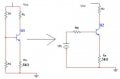# BJT Amplifiers question

#### Digit0001

Joined Mar 28, 2010
100
Hi

The following question can someone explain the first section where they changed the original circuit into thevinin's equivalent. However can someone explain to be what the answers have done?

View attachment question7.doc

This is what i have done:i have changed to thevinin's equivalent and then got stuck because i did not know the values of R1 and R2.

Another question when do we use the resistance reflection rule? Is it only used in a emitter resistance amplifier circuit.

P.S

#### t_n_k

Joined Mar 6, 2009
5,455
The answer seems to be applying a 'rule of thumb' that sets the voltage divider bias network thevenin equivalent resistance to ~10% of the effective DC resistance looking into the base.

#### Digit0001

Joined Mar 28, 2010
100
can anyone answer my second question?

#### t_n_k

Joined Mar 6, 2009
5,455

#### Digit0001

Joined Mar 28, 2010
100
when do we use the resistance reflection rule? Is it only used in a emitter resistance amplifier circuit.

#### Jony130

Joined Feb 17, 2009
5,475
what do you mean by "resistance reflection rule".

#### hgmjr

Joined Jan 28, 2005
9,027
From the context of the question, I suspect that the "reflection rule" referred to here is the reflection of the emitter resistance into the base circuitry.

If my assumption is correct then my answer to that question would be that the "reflection rule" is always applied. Bear in mind that there is an intrinsic emitter resistance that is defined by the equation 0.026/Ie. This resistance is usually so small that when a physical emitter resistor is included, the intrinsic emitter resistance can be conveniently ignored.

hgmjr